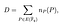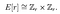# BLS Signatures Part 2 — Key Concepts of Pairings

This is the second part of a two part post explaining in details BLS signatures and how they work.
For part 1 click here

# Point at infinity

A point at infinity is an important concept in elliptic curve math. Its basic definition is a point which is both infinitely high and low in the y direction. We can also imagine the point at infinity as the identity of the group (a special type of element of a set with respect to a binary operation on that set, which leaves any element of the set unchanged when combined with it).

Group points have an order, a number N that if multiplied by the point will “send” the point to infinity.

Example:

E/F101 : y²=x³+x+1, group order #E(Fq)=105, generator(g)=(47,12). Lagrange’s theorem says that to get a point’s order we simply multiply it by the cofactor (see below), where r is the sub-group’s order. A point of order 3 is [105/3](47,12)=(28,8).cofactor

There also exists a point of order 1 which is (47,12) = point at infinity. The point’s order also represents it’s r-torsion group.
R-tortions are extremely important in defining G1 and G2 as we will see below.

# Divisors

At the heart of elliptic curve pairings lies the relationship between the curve and functions which intersect it. A divisor is a way of representing a set of point on curve E:The () around the P indicates it’s a sum in divisor.

Better yet, a divisor is a way to count zeros and infinities of a function on curve E.

Let’s take an example curve, E/F23: y²=x³+5x+7 and the tangent l’: y=4x+20.
We would like to find the divisor (l’).

Substituting l’ in E will result in (4x+20)²=x³+5x+7, simplifying the equation will give us: x³+7x²+6x+21=0 (eq 1)
(remember we are dealing with modular arithmetics so the rules of multiplication, addition and subtraction are a bit different)

We can factorize eq 1 to get (x+21)²(x+11)=0.

We know that a line intersects our curve E in 3 points but since this is a tangent, we will count that point twice. Our tangent intersects E at P(2,5) with multiplicity 2 and at Q(12,1) with multiplicity 1 (note that Q is equal to P).

The divisor of l’ = 2(P)+(Q)-3(O) = 2(P)+P-3(O) with Deg(l’) = 0.

Divisors can be multiplied and divided like functions. For multiplication we simply add up all the divisor points (with multiplicities) and for division we subtract.

Divisors have a concept of degree which is simply adding up the multiplicities.
If (f)=3(P) + 5(R) it’s Deg((F)) = 8.

Divisors can be added together like so:

They also have a concept of support,

With the above in mind, we can think of divisors as sub-groups. Meaning, divisors can be batched up into defined groups depending on their properties.

• Degree zero divisors Div⁰(E) is { D ∈ Div(E), Deg(D)=0}
• If a divisor D on E=(f), f is a function. It’s called a principal divisor, Prin(E).
• Prin(E) ∈ Div⁰(E) ∈ Div(E)

Divisor can be called equivalent if D1 — D2 = (f) ∈ Prin(E), in other words, 2 divisors are equivalent if the difference between them is the zeros and poles of a rational function.

If D is a degree 0 divisor than for some point P it’s equivalent to (P)-(O)

As we will see shortly, the importance of divisors in pairings is crucial as it deals with computing very large degree functions on E, and then evaluating these functions at other divisors.
Evaluating a function f ∈ F_q(E) at a divisor D is defined as:

# Weil Pairing

Let P,Q ∈ E(F_{q^k}[r]) (meaning 2 points on curve E over a field F_{q^k} for the r-torsion group) and D_P, D_Q are degree zero divisors with disjoint supports such that D_P ~ (P)-(O), D_Q ~ (Q)-(O), there exists functions f,g such that (f)=rD_p and (g)=rD_Q.

(multiplying a divisor by a scalar just means multiplying it’s multiplicities)

Weil pairing is defined as:

Essentially, the above means that we take 2 points and map them to another group. This relationship and the way it’s calculated gives us the bi-linearity as described below:

e([a]P, [b]Q) = e(P, [b]Q)^a = e([a]P, Q)^b = e(P, Q)^(ab) = e([b]P, [a]Q)

Let’s see a naive example using the same curve, G_1XG_1=G_T
(example 2.4 page 13 from Steve Diaz’s paper).

Let S, T ∈ E[n]. Let D_S and D_T be divisors of degree 0 such that sum(D_S) = S and sum(D_T ) = T and such that D_S and D_T have no points in common. Let f_S and f_T be functions such that div(f_S ) = nD_S and div(f_T ) = nD_T .

Find e(S,T)

A natural choice of divisors is D_S =[S]−[∞], D_T =[T+R]−[R].
E/F_7: y²=x³+2 (eq 2)
P=(0,3),T=(5,1) such that D(0,3) =[(0,3)]−[O],
D(5,1)=[((5,1)+(6,1))]−[(6,1)]=[(3,6)]−[(6,1)].
f: y-3 and g: (4x-y+1)/5x-y-1). D(f)=3D_P and D(g)=3D_T
* For finding f and g we can use Miller’s algorithm

Our next step is to calculate f(D_Q), g(D_P).
f(D_T)=f(3,6)/f(6,1)=(6-3)/(1–3)=2 (mod 7)
g(D_P)=4 (mod 7)

The way we calculate the point to infinity is by transferring to projective coordinates and treating the point to infinity as (0,1,0). Our pairing result is e(S,T)=4/2=2 (mod 7)

Bilinearity

Now comes the cool part. The way we evaluate divisor points at functions treats the multiplicity of a point (in the divisor) as an exponent, this means that if we would have done this example for P, the result would have been e(S,T)². That’s some cool bilinearity!

The example above is of-course not very secure, usually the curve needs to be over a very large field and the r-torsion group needs to be very big as well.
This leads to an issue as D_P, D_T need to have disjoint support which can’t be found in F_q anymore. That is why most secure implementations of pairings do not use symmetric groups (G_1XG_1) but rather group extensions (think complex numbers).

Group Extensions

From the example above we’ve learned that divisors used in a weil pairing should come from disjoint groups, that is, no points in common.
If our selected r-torsion group is small, we might find 2 cyclic groups in E/F_q but if it’s big we won’t be able to.

From eq. 2 above we have: E/F_7: y²=x³+2, #E(F_7) = 9 (E has 9 points).
Luckily we had 2 distinct order 3 groups:
* (0,3), (0,4) and O (inf)
* (5,1), (5,6) and O (inf)
This enabled us to calculate e((0,3), (5,1)) as they are 2 disjoint groups of order 3.

If E was over F_11 instead and we would have chosen to calculate our pairing for points in the 12th torsion (like point 1,5) than we could not have found 2 disjoint groups of order 12 in E. What can we do?
The answer is extending the field ovre which E is set on, using complex numbers.
For example: E/F_7691: y²=x³+1. Suppose we construct E such that it’s over F_q² = F_q(i) where i²+1=0, we can now represent points on a curve as complex numbers, for example Q = (633i + 6145, 7372i + 109) which is of prime order 641, just like P = (2693, 4312) ∈ E(F_q).

As it turns out, we can actually calculate how big is an r-torsion group.R. M. Avanzi, H. Cohen, C. Doche, G. Frey, T. Lange, K. Nguyen, and F. Vercauteren. The Handbook of Elliptic and Hyperelliptic Curve Cryptography and J. H. Silverman. The Arithmetic of Elliptic Curves (2nd Edition).

This means that in general, #E[r] = r² and that the r-torsion consists of r+1 cyclic groups of order r. Notice that only some of the points are actually in F_q.

# Summary

BLS signatures are based on a concept called pairing which presents bilinearity between it’s components. Those are just fancy words for saying that we can apply, for example, some multiplication on one of the components of the pairing and the result will be that base pairing result to the a.

This fact enables us to evaluate, via pairings, digital signatures. The nice characteristics of BLS signatures are that they are aggregatable and deterministic which opens up a lot of possibilities.

Two of those possibilities are:

• eth 2.0: as it is built today, using committee which aggregate signatures. This enables finality within 2 epochs (~13min)
• staking pools: as the signatures are deterministic and aggregatable it makes them ideal to use within an MPC setting of staking pools.

Thank you Leonid Beder, Kobi Gurkan, Omer Shlomovits, Guy Corem and Barak for helping and peer reviewing this article and providing valuable feedback.

CEO @ bloxstaking.com and blox.io. Developing trustless staking products for eth2.0.

## More from Alon Muroch

CEO @ bloxstaking.com and blox.io. Developing trustless staking products for eth2.0.